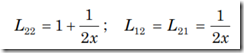### Electrical Machines–1–April / May 2010 Question Paper

Anna University

B.E./B.Tech. DEGREE EXAMINATION, APRIL/MAY 2010.

Fourth Semester

Electrical and Electronics Engineering EE2251 — ELECTRICAL MACHINES – I

(Regulation 2008)

Time: Three hours

Maximum: 100 Marks

PART A — (10 × 2 = 20 Marks)

1. Define Torque.

2. How is emf induced dynamically?

3. Give the principle of transformers.

4. What are the conditions for parallel operation of transformers?

5. In a linear system prove that field energy and co-energy are equal.

6. Write an expression for the stored energy in the magnetic field.

7. What are the basic magnetic field effects that result in the production of mechanical forces?

8. What are the assumptions made to determine the distribution of coil mmf?

9. What is armature reaction?

10. What are the methods of speed control in DC motor?

PART B — (5 × 16 = 80 Marks)

11. (a) Discuss in detail the following: (i) B-H relationship

(ii) Leakage flux

(iii) Fringing

(iv) Stacking factor. (4 × 4 = 16)

Or

(b) (i) Derive an expression for energy density in the magnetic field. (6)

(ii) Explain in detail ‘‘Eddy – current loss’’. (5)

(iii) The total core loss of a specimen of silicon steel is found to be 1500 W at 50 Hz. Keeping the flux density constant the loss becomes 3000 W when the frequency is raised to 75 Hz. Calculate separately the hysteresis and eddy current loss at each of there frequencies. (5)

12. (a) (i) Draw the equivalent circuit of single phase transformer and draw the necessary phasor diagram under load

(1) Resistive (2) Inductive (3) Capacitive. (8)

(ii) Explain in detail the tests required to obtain the equivalent circuit parameters of transformer. (8)

Or

(b) (i) Explain in detail the various types of three phase transformer connection. (10)

(ii) Prove that amount of copper saved in auto transformer is (1 – K)times that of ordinary transformer. (6)

13. (a) (i) Derive an expression for mechanical force in terms of field energy. (8)

(ii) Discuss the flow of energy in electromechanical devices in detail. (8)

Or

(b) (i) Derive an expression for torque in case of a multiply excited magnetic field system. (8)

(ii) Two coupled coils have self and mutual inductance ofover a certain range of linear displacement x. The first coil is excited by a constant current of 20A and the second by a constant current of –10A.

Find:

(1) Mechanical work done if x changes from 0.5 to 1 m.

(2) Energy supplied by each electrical source in part (a).

(3) Change in field energy. (8)

14. (a) Explain in detail the basic concept of a synchronous generator with a neat diagram and the necessary space wave form. (16)

Or

(b) (i) Discuss the basic concept of emf generation in a DC machine in detail. (8)

(ii) What is MMF space wave of a single coil and in a distributed winding? (8)

15. (a) (i) Explain armature reaction and commutation in detail. (8)

(ii) Draw the

(1) OCC characteristics of DC generator and (4)

(2) External characteristics of DC generator. (4)

Or

(b) (i) Explain in detail the various methods of speed control in DC motor. (8)

(ii) What are the various starting methods of DC motor? Explain any one method. (8)